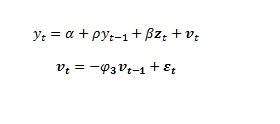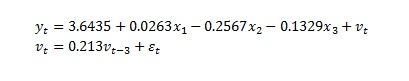## Forecast Autoregressive Error Model with PROC ARIMA

I'm wondering how to forecast a published autoregressive error model, estimated in PROC AUTOREG, using PROC ARIMA. I have some data I would like to a monthly time series, for example:

``````data inputs;
input month y var1 var2 var3 var4
datalines
1    200    150    3    6    8
2    300    200    8    4    8
3    270    300    4    3    1
4    250    270    7    4    5
;``````

Where var 1 is a lagged dependent variable. Suppose I estimate the following model

``````proc autoreg data=some_data outest=params;
model y = var1 var2 var3 var4 /
lagdep=var1 method=ml nlag=12 backstep;
output out=model p=yhat r=residuals;
run;``````

where var1 is a lagged dependent variable, and var2-var4 are some explanatory variables. The final model contains an AR(3) parameter for the model of the error series. If this model was estimated 1 year ago, and I want to produce a multistep forecast of the model today, I might use PROC ARIMA coupled with the "Noest" option to suppress estimation of the model:

``````proc arima data=some_forecast_data plots(unpack)=forecast(all);
identify var=y crosscorr(=var2 var3 var4);
estimate p=(1) ar=0.2343 mu=7.9534
input=(/(1)var2 /(1)var3 /(1)var4)
initval=(-0.564\$/(0.2343)var2
0.0041\$/(0.2343)var3
0.1753\$/(0.2343)var4
) noest;
forecast id=month interval=month lead=25 out=forecast_data nooutall;
run;
``````

But this does not include the AR(3) parameter of the autoregessive error model. How can I include the additional AR terms for the autoregressive error model? How does PROC ARIMA handle the model of the residual series if the model is not re-estimated?

1 ACCEPTED SOLUTION

Accepted Solutions

## Re: Forecast Autoregressive Error Model with PROC ARIMA

In that case, the term associated with var1 must become part of the AR spec.  In fact, in your first spec you had tried something like that already.  Anyway, here is what it would look like (you might have to play with the signs of the coefficients so that they conform to the ARIMA syntax convention):

proc arima data=test plots(unpack)=forecast(all);
identify var=y cosscorr=(var2 var3 var4);
estimate p=(1)(3) ar=0.0422 0.213 mu=3.80403
input=(/1 var2 /1 var3 /1 var4)
initval=(0.0263\$/(0.0422)var2
-0.2567\$/(0.0422)var3
-0.1329\$/(0.0422)var4) noest;
forecast id=month interval=month lead=25 out=forecast_data nooutall;
run;

Here mu=3.6435/(1-0.0422)

11 REPLIES 11

## Re: Forecast Autoregressive Error Model with PROC ARIMA

I am not quite sure what you need.  Can you please give me the precise model that you want to specify using ARIMA?  What are AR and MA orders, differencing orders, response variable, and predictors, etc.

Note, when you specify the NOEST option, you must specify the values of all the parameters (except the error variance).

PS.  I am traveling overseas.  I will try to answer your question after reaching my destination in about two days.  If you need an answer sooner, you can try the Tech Support.

## Re: Forecast Autoregressive Error Model with PROC ARIMA

I estimated the following model in PROC AUTOREG using maximum likelihood (method=ml), specifying nlag=12 and the backstep option to automtically select the autoregressive order.where y_(t-1) is a lagged dependent variable and z_t is an array of explanatory variables. Because this model was estimated with nlag and backstep, SAS automatically selects the autoregressive error model for the noise series.

Finally, the estimated model is:This model was estimated approx 1 yr ago, and I want to forecast this model in PROC ARIMA without re-estimating the model, thus using the NOEST option. I'm wondering how to forecast this model, including the AR(3) parameter for v_t, estimated by PROC AUTOREG using PROC ARIMA without re-estimating the model?

## Re: Forecast Autoregressive Error Model with PROC ARIMA

Based on your final model (treating x1-x3 as var2-var4), I would modify your syntax as follows:

proc arima data=test plots(unpack)=forecast(all);
identify var=y cosscorr=(var2 var3 var4);
estimate p=(3) ar=0.213 mu=3.6435
initval=(0.0263 var2 -0.2567 var3 -0.1329 var4) noest;
forecast id=month interval=month lead=25 out=forecast_data nooutall;
run;

Note that your AR model has just one term of order 3, which is signified by p=(3).

## Re: Forecast Autoregressive Error Model with PROC ARIMA

Forgot to include the input part:

proc arima data=test plots(unpack)=forecast(all);
identify var=y cosscorr=(var2 var3 var4);
estimate p=(3) ar=0.213 mu=3.6435

input=(var2 var3 var4)
initval=(0.0263 var2 -0.2567 var3 -0.1329 var4) noest;
forecast id=month interval=month lead=25 out=forecast_data nooutall;
run;

## Re: Forecast Autoregressive Error Model with PROC ARIMA

Thanks. What about the lagged dependent variable? Shouldn't that be specified as an AR(1)?

## Re: Forecast Autoregressive Error Model with PROC ARIMA

I forgot to include that in the above model. My fault. There proper model should be:

Y_t = 3.6435 + 0.0422 Y_(t-1) + 0.0263 x_1 -0.2567 x_2 - 0.1329 x_3 + v_t,

with the same AR(3) autoregressive error structure.

## Re: Forecast Autoregressive Error Model with PROC ARIMA

As far as just the spec is concerned, ARIMA does not know that var1 is a lagged y value.  Its spec will be like other regressors (V2, V3, and V4).  I find your model odd.  You will not have future values of var1 in the forecast period and then the forecasts cannot be produced.  Specifying an AR term does not mean including lagged y-values as regressors.

## Re: Forecast Autoregressive Error Model with PROC ARIMA

However, it is possible to produce multistep forecasts for dynamic models whereby the forecasted values are produced using lagged forecasted values rather than lagged actual values (i.e. this can be done in PROC SIMLIN). However, with the model of the error series (in this case AR3), is it possible to forecast this in PROC SIMLIN or PROC ARIMA? Thanks!

## Re: Forecast Autoregressive Error Model with PROC ARIMA

In that case, the term associated with var1 must become part of the AR spec.  In fact, in your first spec you had tried something like that already.  Anyway, here is what it would look like (you might have to play with the signs of the coefficients so that they conform to the ARIMA syntax convention):

proc arima data=test plots(unpack)=forecast(all);
identify var=y cosscorr=(var2 var3 var4);
estimate p=(1)(3) ar=0.0422 0.213 mu=3.80403
input=(/1 var2 /1 var3 /1 var4)
initval=(0.0263\$/(0.0422)var2
-0.2567\$/(0.0422)var3
-0.1329\$/(0.0422)var4) noest;
forecast id=month interval=month lead=25 out=forecast_data nooutall;
run;

Here mu=3.6435/(1-0.0422)

## Re: Forecast Autoregressive Error Model with PROC ARIMA

Thanks for the clarification. That confirmed what I previously thought. Quick follow up: Is it possible to forecast that type of dynamic autoregressive error model in PROC SIMLIN, or any other SAS procedures besides PROC ARIMA? It's a cumbersome syntax for what is really a quite simple model.

## Re: Forecast Autoregressive Error Model with PROC ARIMA

I agree about the tediousness of ARIMA syntax.  Unfortunately, I cannot think of any other option for you for such a spec.

Discussion stats
• 11 replies
• 2146 views
• 0 likes
• 2 in conversation